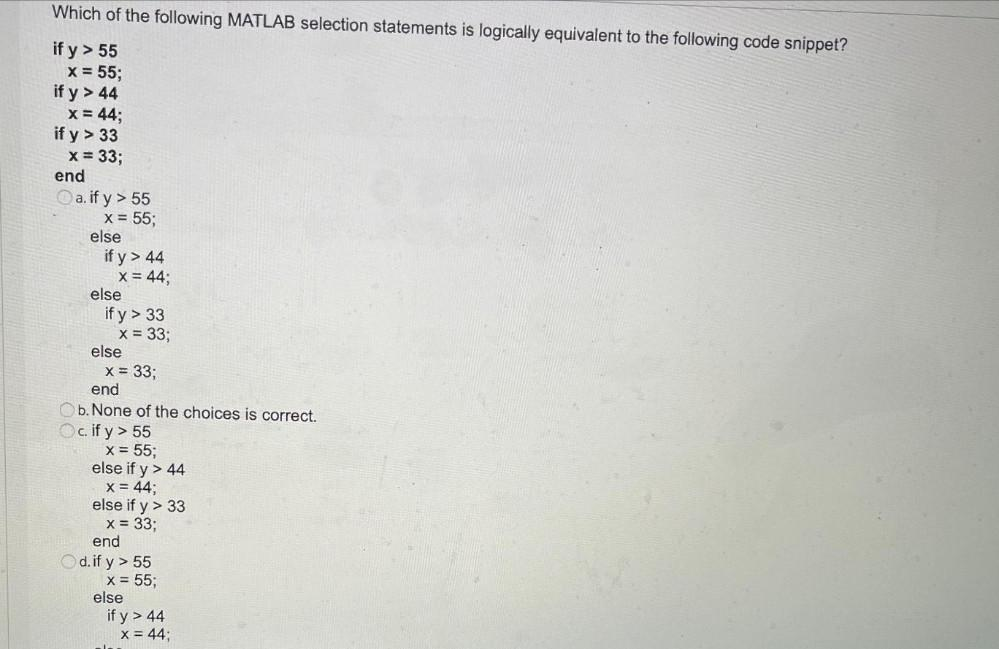Question:

# Which of the following MATLAB selection statements is logically equivalent to the following code snippet? if y > 55 x = 55; if yWhich of the following MATLAB selection statements is logically equivalent to the following code snippet? if y > 55 x = 55; if y > 44 x = 44; if y > 33 x = 33; end a. if y> 55 X = 55; else if y> 44 x = 44; else if y> 33 x = 33; else x = 33; end b. None of the choices is correct. c. if y> 55 x = 55; else if y> 44 x = 44; else if y> 33 x = 33; end d. if y> 55 x = 55; else if y > 44 x = 44;